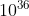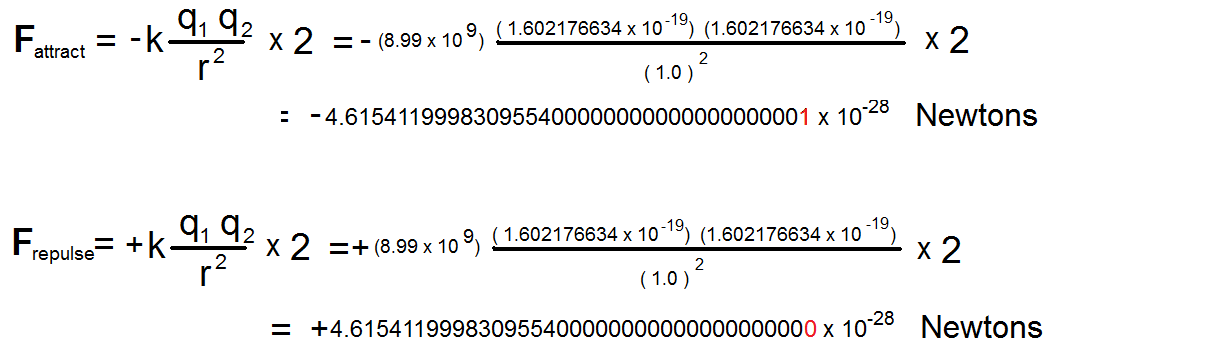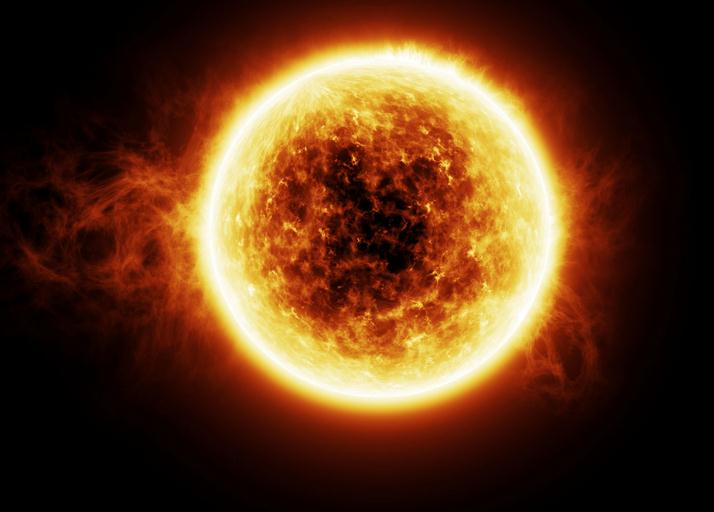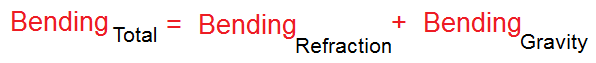Science Forums

# 7 Reasons To Abandon Quantum Mechanics-And Embrace This New Theory

## Recommended Posts

Quote

Devin553344 said:     Like charges repel while opposite charges attract. I think you missed the basics here. Gravity is not the electrical force. Atoms are neutral. And even if they weren't perfectly neutral it still wouldn't account for gravitation where all matter attracts in the universe. Charge is also invariant while mass is not considering relativity

Edited by andrewgray
• Replies 380
• Created

#### Popular Posts

October 2012 Update Edit: This forum is about our new Intermittent Electron Theory that has been recently published in Physics Essays: The Theory of Intermittent Electrons

Many of your reasons for abandoning quantum mechanics actually do have explanations. Consider your "photoelectric paradox." The first thing to realize is that this treatment of the photoelectric eff

You are correct, though the radiation equation WOULD imply spherical radiation for this system. The solution to this paradox is the simple fact that a blinking electron is incompatible with maxwell's

Posted (edited)

Devin,

Quote

Like charges repel while opposite charges attract.

Correct.

Quote

Gravity is not the electrical force.

Correct.

What gravity is... is a Residual Electrical Force.  That is, (between 2 bodies) the sum of the electrical attractive forces plus the sum of the electrical repulsive forces is very close to zero, but not quite!   The sum of the electrical attractive forces plus the sum of the electrical repulsive forces is close to zero by one part in!  That is unimaginably close to zero!  But that extremely, extremely small left-over-force is enough to make gravity.

We cannot say what the residual charges are.  Only that the sum of the electical attractive forces plus the electrical repulsive forces is extremely, extremely close to zero, but ever so slightly attractive!   And by symmetry, that would make anti-matter gravitationally repulsive to matter, contradicting Einstein.

Devin,

Quote

Charge is also invariant

Correct.

So is the "gravitational mass" invariant, since large gravitational bodies in the universe move "slowly" relativity-wise.  The gravitational mass would be proportional to the number of proton-electron pairs a body possessed (remembering to count each of its neutrons as one proton and one electron!)

So that means that the gravitational force is indeed a vector force... so that means that we will have to deal with Einstein, his equivalence principle, Spacetime Curvature, and his General Relativity!  Coming!

Andrew Ancel Gray

Edited by andrewgray
##### Share on other sites
On 10/2/2020 at 1:28 PM, andrewgray said:

Devin,

Correct.

Correct.

What gravity is... is a Residual Electrical Force.  That is, (between 2 bodies) the sum of the electrical attractive forces plus the sum of the electrical repulsive forces is very close to zero, but not quite!   The sum of the electrical attractive forces plus the sum of the electrical repulsive forces is close to zero by one part in!  That is unimaginably close to zero!  But that extremely, extremely small left-over-force is enough to make gravity.

We cannot say what the residual charges are.  Only that the sum of the electical attractive forces plus the electrical repulsive forces is extremely, extremely close to zero, but ever so slightly attractive!   And by symmetry, that would make anti-matter gravitationally repulsive to matter, contradicting Einstein.

Devin,

Correct.

So is the "gravitational mass" invariant, since large gravitational bodies in the universe move "slowly" relativity-wise.  The gravitational mass would be proportional to the number of proton-electron pairs a body possessed (remembering to count each of its neutrons as one proton and one electron!)

So that means that the gravitational force is indeed a vector force... so that means that we will have to deal with Einstein, his equivalence principle, Spacetime Curvature, and his General Relativity!  Coming!

Andrew Ancel Gray

I think you're missing the point, how is it that the sun, planets, moons all attract, but none repel, so your idea is that there is some charge to the sun, planets and moons. Have you worked out any math or calculations? Probably not cause then you would see that your idea is invalid.

##### Share on other sites
Posted (edited)

I'm glad you asked this question, Devin.  You see, it is not residual charge that I am talking about.  It is residual electric force.  There is a big difference.  What I am saying is that atoms are completely made up of protons and electrons (each neutron is a proton and electron combo).  Then, between two bodies that have the save number of protons and electrons (neutral), the attractive electrical forces are ever, ever, ever, ever so slightly greater than the repulsive electrical forces by one part in!  This says nothing about charge.  We are only dealing with attractive and repulsive electrical force.  the two bodies  have the same number of positive and negative charges.  But the attractive electrical forces are ever so slightly larger than the repulsive electrical forces.

Calculations?  OK. An example:

Take two hydrogen atoms, A and B ,  separated by one meter.  They each have one electron and one proton. Now the attractive electrical forces between them are the e-p and the p-e electrical forces.  The repulsive electrical forces would be the e-e and the p- electrical forces.

The attractive and repulsive electrical forces would be about:The magnitude of these two numbers are experimentally the same!  They only differ by one part in(see the last red digit).  You could not measure this difference experimentally!  But this "teensy weensy tee-niney" difference in magnitude is enough to give the two hydrogen atoms a gravitational attraction!

One last thing.  Remember, the proton and electron are completely different "animals".  The proton is more than a thousand times more massive than the electron.  They both pulsate.  They probably pulsate differently...

So... it does not seem unreasonable, that out to some decimal place, the attractive and repulsive electrical forces are different.  Devin, do you believe they are the same out past 36 decimal places? Do you believe they are the same out past a 1000 decimal places?  Out past a 10,000 decimal places?  They are "different animals".  In my mind, no one in their right mind would believe this! (Perhaps electron-positron attractive/repulsive forces go out more decimal places the same, but not electron-proton ones!)

Andrew Ancel Gray

PS.  Now on with Gravity?

PSS.  There is gravitational repulsion!  By symmetry, antimatter would repel "regular matter" gravitationally, not attract, contradicting Einstein.

So Devin, do you at least understand what I am saying (but maybe not agree?) ???

Edited by andrewgray
##### Share on other sites
Posted (edited)
On 10/5/2020 at 11:04 AM, andrewgray said:

o... it does not seem unreasonable, that out to some decimal place, the attractive and repulsive electrical forces are different.

No, it seems reasonable to expect them to be the exact same. You need to be able to supply a reason why not. All the teeny, tiny, teeniest rhetoric hasn't done that.

Would you not be looking for this kind of response on a science forum?

For example: One measured nautical mile is exactly the same length as all other measured nautical miles.

Edited by montgomery
##### Share on other sites
Posted (edited)
On 10/5/2020 at 11:04 AM, andrewgray said:

I'm glad you asked this question, Devin.  You see, it is not residual charge that I am talking about.  It is residual electric force.  There is a big difference.  What I am saying is that atoms are completely made up of protons and electrons (each neutron is a proton and electron combo).  Then, between two bodies that have the save number of protons and electrons (neutral), the attractive electrical forces are ever, ever, ever, ever so slightly greater than the repulsive electrical forces by one part in!  This says nothing about charge.  We are only dealing with attractive and repulsive electrical force.  the two bodies  have the same number of positive and negative charges.  But the attractive electrical forces are ever so slightly larger than the repulsive electrical forces.

Calculations?  OK. An example:

Take two hydrogen atoms, A and B ,  separated by one meter.  They each have one electron and one proton. Now the attractive electrical forces between them are the e-p and the p-e electrical forces.  The repulsive electrical forces would be the e-e and the p- electrical forces.

The attractive and repulsive electrical forces would be about:

The magnitude of these two numbers are experimentally the same!  They only differ by one part in(see the last red digit).  You could not measure this difference experimentally!  But this "teensy weensy tee-niney" difference in magnitude is enough to give the two hydrogen atoms a gravitational attraction!

One last thing.  Remember, the proton and electron are completely different "animals".  The proton is more than a thousand times more massive than the electron.  They both pulsate.  They probably pulsate differently...

So... it does not seem unreasonable, that out to some decimal place, the attractive and repulsive electrical forces are different.  Devin, do you believe they are the same out past 36 decimal places? Do you believe they are the same out past a 1000 decimal places?  Out past a 10,000 decimal places?  They are "different animals".  In my mind, no one in their right mind would believe this! (Perhaps electron-positron attractive/repulsive forces go out more decimal places the same, but not electron-proton ones!)

Andrew Ancel Gray

PS.  Now on with Gravity?

PSS.  There is gravitational repulsion!  By symmetry, antimatter would repel "regular matter" gravitationally, not attract, contradicting Einstein.

So Devin, do you at least understand what I am saying (but maybe not agree?) ???

Andrew, it would appear that in order to understand electric force, you must first understand how it works. Electric force is a function of electric charge. Without electric charge force does not exist. In fact without an imbalance in charge, there is no force to even consider. Which is why I cannot consider your idea.

Not to mention the fact that the electric escape energy (force) is subtracted or added to the mass of the parts of the electrons and protons. Which is why there is no additional energy floating around either. So there's no force for your idea. Calculations? Look at hydrogen, the force of the electron is removed from the mass of the electron and proton, this is the basic principle everyone accepts.

Keep working on it, but you're headed in the wrong direction here.

Edited by devin553344
##### Share on other sites
On 10/6/2020 at 3:39 PM, montgomery said:

No, it seems reasonable to expect them to be the exact same. You need to be able to supply a reason why not. All the teeny, tiny, teeniest rhetoric hasn't done that.

Would you not be looking for this kind of response on a science forum?

For example: One measured nautical mile is exactly the same length as all other measured nautical miles.

Montgomery,

Well, if an experimenter cannot measure a value past 10 decimal places, then the value of the 36th decimal place can be hypothesized to be anything!  This is just plain philosophical logic.  So, I am hypothesizing that the sum of the repulsive electrical forces plus the sum of the attractive electrical forces between proton/electron pairs is slightly skewed towards the attractive side by one part in, leaving a residual force of gravity.  You do not have to agree, but I can hypothesize this difference no problem!   I believe it is justified just because we have a force of gravity, and because the electron and proton are not matter-antimatter pairs (they are "different animals").  I believe the existence of gravity makes it more likely that the sum of the electrical forces are skewed attractive!

And Devin, I understand how electric force works just fine.   And yes, what you are referring to is the binding energy.  The neutron is a composite of a proton and an electron with a binding energy that is more than hydrogen's, so the neutron's mass is less than hydrogen's.  The physics community has had the mass of the neutron incorrect for decades because of the incorrect use of "fauxton" gamma ray "light particles" in the mass equation.  No!

So no more delays.  On with gravity!

If gravity is indeed a force, then we will have to deal with Einstein.  So let's do that.  First, we deal with his Equivalence Principle!

Einstein's original equivalence principle essentially states that

Quote

"locally, one cannot tell the difference between a gravity field and a frame that is undergoing acceleration".

But this is not quite correct!  So here is how WE are going to alter Einstein's equivalence principle!

Einstein's equivalence principle will now state that

Quote

locally, one cannot tell the difference between masses in motion in a gravity field and masses in motion in a frame that is undergoing acceleration!

Notice that we have added the terms "masses in motion" to the equivalence principle.  That is because since gravity is a force, and since the gravitational mass and the inertial mass cancel out of Newton's Law (for example:)then acceleration is independent of mass, and all masses follow the same path in a gravity field.  OK, so far so good...

But this is NOT true for light!  For light, one can tell the difference.  As a matter of fact, this is how one tells the difference between a gravity field and a frame experiencing acceleration!  Light bends in the accelerating frame and not in a gravity field.

Quote

"Light will bend in a frame experiencing acceleration, but it will not bend in a gravity field!"

OK.  I know.  I know.  The "bending around near the sun experiments using a solar eclipse" needs to be addressed.  I know. This is a dirty tale of confirmation bias and almost scientific misconduct!  Einstein predicted a gravitational bending of light around the sun.  OK, here is the sun and the sun's atmosphere:So if we wanted to calculate how much light bends around the sun, we would calculate:Well, it turns out that the amount of bending measured is fairly close to the Gravity Bending term by itself!  Oh, no!  But that did not stop the confirmation bias!  So all they had to do was zero out the Refraction Bending, like this!! :Then... they can claim the light bending agrees with Einstein!  Really?  Just look at the solar atmosphere!  There is going to be lots of bending due to that!  Heck, the Earth's atmosphere bends light by 3 degrees!  Putting in a "0" for refraction bending so that the bending value can agree with Einstein's is a typical story of confirmation bias and should not be tolerated.  IF Einstein would have been correct, then the bending would have BEEN GREATER THAN WHAT WAS CALCULATED FOR THE REFRACTION, and we would expect to see a value GREATER THAN EINSTEIN's!  But we do not.  So our modified Equivalence Principle is safe, and we see that the bending of light around the sun is most definitely characterized by this equation:Light bending around the sun is due to the refraction from the sun's atmosphere!

Next:  The Pound and Rebka redshift experiment.  Wait till you find out what THEY did!

Edited by andrewgray

## Join the conversation

You can post now and register later. If you have an account, sign in now to post with your account.×   Pasted as rich text.   Paste as plain text instead

Only 75 emoji are allowed.

×   Your previous content has been restored.   Clear editor

×   You cannot paste images directly. Upload or insert images from URL.